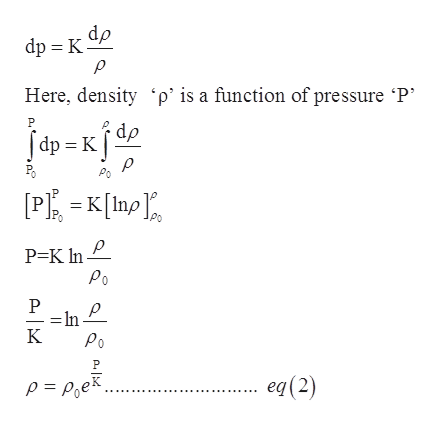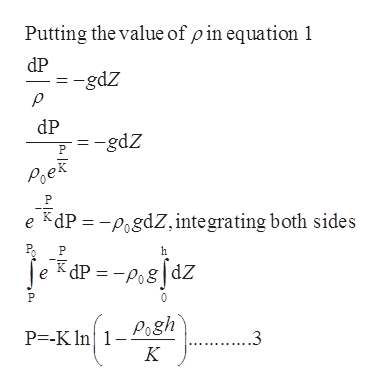# Fluid MechanicsLast fall, researchers using a manned submarine discovered coral reefs at unprecedented ocean depths of 1/2 mile, of the coast of South Carolina. Find the hydrostatic pressure at that depth. Find the fractional changes in density (drho/rho) and in volume (dV/V) for the seawater at that depth. The density of seawater at the surface of the ocean is 1025kg/m3, and the bulk modulus of elasticity of seawater is 2.34*109 Pa.

Question

Fluid Mechanics

Last fall, researchers using a manned submarine discovered coral reefs at unprecedented ocean depths of 1/2 mile, of the coast of South Carolina. Find the hydrostatic pressure at that depth. Find the fractional changes in density (drho/rho) and in volume (dV/V) for the seawater at that depth. The density of seawater at the surface of the ocean is 1025kg/m3, and the bulk modulus of elasticity of seawater is 2.34*109 Pa.

check_circleExpert Solution
Step 1

The general equation for the hydrostatic pressure is given as follows:

Step 2

The equation for the bulk modulus of elasticity is as follows:help_outlineImage Transcriptionclosedp dp K Here, density p' is a function of pressure P P dp p Po PK[p P=K ln Po P In K Po eq (2) p = Poe fullscreen
Step 3help_outlineImage TranscriptionclosePutting the value of pin equation 1 dP -gdZ dP =-gdZ P PoeR KdP -PogdZ, integrating both sides e dPPodz e 1- Pogh K P=-K ln ..3 fullscreen

### Want to see the full answer?

See Solution

#### Want to see this answer and more?

Solutions are written by subject experts who are available 24/7. Questions are typically answered within 1 hour*

See Solution
*Response times may vary by subject and question
Tagged in

### Fluid Mechanics